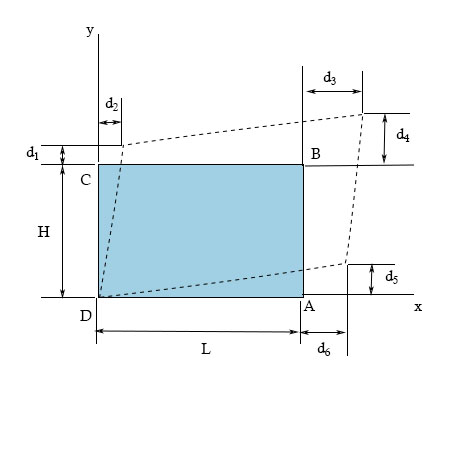# The plastic distorts as shown by the dashed lines.(Figure 1) The dimensions are L=500 mm, H=340...

## Question:

The plastic distorts as shown by the dashed lines. (Figure 1)

The dimensions are {eq}L=500 mm, H=340 mm, d_1=3 mm, d_2=5 mm,d_3=14 mm, d_4=9 mm, d_5=1 mm, d_6=4 mm {/eq}.

Part A

Determine the shear strain {eq}\tau_{xy} {/eq} at corner A. Express your answer to three significant figures and include appropriate units.

Part B

Determine the shear strain {eq}\tau_{xy} {/eq} at corner B. Express your answer to three significant figures and include appropriate units.## Shear strain

The shear strain can be defined as the ratio of deformed to original dimensions. In the shear strain, the amount of deformation is perpendicular to a given line rather than parallel to it.

Become a Study.com member to unlock this answer! Create your account

Given Data:

• The length of the section is {eq}L = 500\;{\rm{mm}} {/eq}.
• The height of the section is {eq}H = 340\;{\rm{mm}} {/eq}.
• The deflection at...